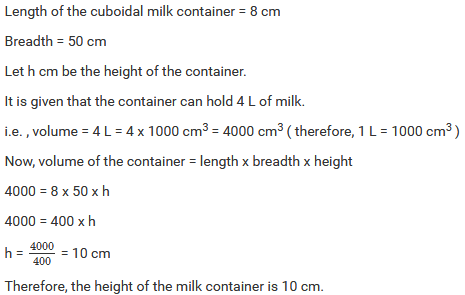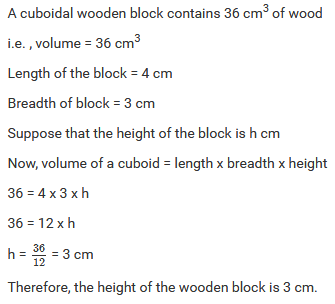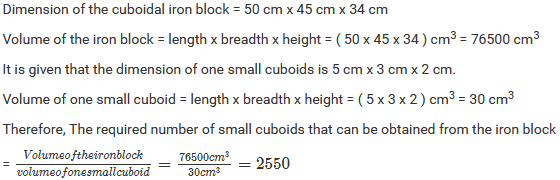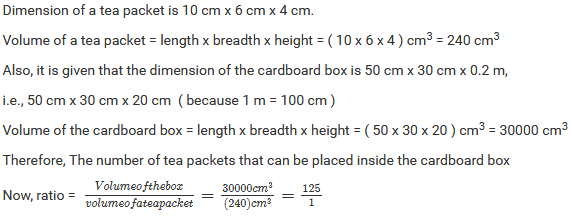×#### Thank you for registering.

One of our academic counsellors will contact you within 1 working day.

Click to Chat

1800-1023-196

+91-120-4616500

CART 0

• 0

MY CART (5)

Use Coupon: CART20 and get 20% off on all online Study Material

ITEM
DETAILS
MRP
DISCOUNT
FINAL PRICE
Total Price: Rs.

There are no items in this cart.
Continue Shopping• Complete JEE Main/Advanced Course and Test Series
• OFFERED PRICE: Rs. 15,900
• View Details

```Mensuration II (Volumes and Surface Areas of a Cuboid and a Cube) Exercise 21.1

Question: 1

Find the volume of a cuboid whose:

i) Length = 12 cm, breadth = 8 cm and height = 6 cm

ii) length = 1.2 m, breadth = 30 cm ,height = 15 cm

iii)  length = 1.5 dm, breadth = 2.5 dm, height = 8 cm

Solution:

i) In the given cuboid, we have:

Length = 12 cm, breadth = 8 cm and height = 6 cm

Therefore,  Volume of the cuboid = length x breadth x height =12 x 8 x 6 = 576 cm3

Therefore,  Volume of the cuboid = 576 cm3

ii) In the given cuboid, we have :

length = 1.2 m = 1.2 x 100 cm ( 1 m = 100 cm ) = 120 cm

height = 15 cm

Therefore, Volume of the cuboid = length x breadth x height = 120 x 30 x 15 = 54000 cm3

Therefore, Volume of the cuboid = 54000 cm3

iii) In the given cuboid, we have :

length = 1.5 dm = 1.5 x 10 ( 1 dm = 10 cm ) = 15 cm

breadth = 2.5 dm =2.5 x 10 cm =25 cm

height = 8 cm

Therefore, Volume of cuboid = length x breadth x height = 15 x 25 x 8 = 3000 cm3

Therefore, Volume of cuboid = 3000 cm3

Question: 2

Find the volume of cube whose side is:

i) 4 cm

ii) 8 cm

iii) 1.5 dm

iv) 1.2 m

v) 25 mm

Solution:

i) The side of the given cube is 4 cm

Therefore, Volume of the cube = ( side )3 = ( 4 )3 = 64 cm3

Volume of the cube = 64 cm3

ii) The side of the given cube is 8 cm

Therefore, Volume of the cube = ( side )3 = ( 8 )3 = 512 cm3

Volume of the cube = 512 cm3

iii) The side of the given cube is 1.5 dm = 1.5 dm x 10 cm = 15 cm

Therefore, Volume of the cube = ( side )3 = ( 15 )3 = 3375 cm3

Volume of the cube = 3375 cm3

iv) The side of the given cube is 1.2 m = 1.2 m x 100 = 120 cm

Therefore, Volume of the cube = ( side )3 = ( 120 )3 = 1728000 cm3

Volume of the cube = 1728000 cm3

v) The side of the given cube is 25 mm = 25 mm x 0.1 = 2.5 cm

Therefore, Volume of the cube = ( side )3 = ( 2.5 )3 = 15.625 cm3

Volume of the cube = 15.625 cm3

Question: 3

Find the height of a cuboid of volume 100cm3, whose length and breadth are 5 cm and 4 cm respectively.

Solution:Question: 4

A cuboidal vessel is 10 cm long and 5 cm wide. How high must it be made to hold 300 cm3 of a liquid?

Solution:

Let h cm be the height of the cuboidal vessel.

Given : Length = 10 cm

Volume of the vessel = 300 cm3

Now, volume of a cuboid = length x breadth x height

300 = 10 x 5 x h

300 = 50 x hQuestion: 5

A milk container is 8 cm long and 50 cm wide. What should be its height so that it can hold 4 liters of milk?

Solution:Question: 6

A cuboidal wooden block contains 36 cm3 wood. If it be 4 cm long and 3 cm wide, find its height.

Solution:Question: 7

What will happen to the volume of the cube , if its edge is :

i) Halved

ii) Trebled?

Solution:Question: 8

What will happen to the volume of cuboid if its :

i) Length is doubled, height is same and breadth is halved?

ii) length is doubled, height is doubled and breadth is same?

Solution:Question: 9

Three cuboids of dimensions 5 cm x 6 cm x 7 cm , 4 cm x 7 cm x 8 cm and 2 cm x 3 cm x 13 cm are melted and a cube is made. Find the side of cube.

Solution:Question: 10

Find the weight of a solid rectangular iron piece of size 50 cm x 40 cm x 10 cm, if 1 cm3 of iron weighs 8 gm.

Solution:Question: 11

How many wooden cubical blocks of side 25 cm can be cut from a log of wood of size 3 m by 75 cm by 50 cm, assuming that there is no wastage?

Solution:Question: 12

A cuboidal block of silver is 9 cm long, 4 cm broad and 3.5 cm in height. From it, beads of volume 1.5 cm3 each are to be made. Find the number of beads that can be made from the block.

Solution:Question: 13

Find the number of cuboidal boxes measuring 2 cm by 3 cm by 10 cm which can be stored in a carton whose dimensions are 40 cm, 36 cm, and 24 cm.

Solution:Question: 14

A cuboidal block of solid iron has dimensions 50 cm, 45 cm and 34 cm. How many cuboids of size 5 cm by 3 cm by 2 cm can be obtained from this block? Assume cutting causes no wastage.

Solution:Question: 15

A cube A has side thrice as long as that of cube B. What is the ratio of the volume of cube A to that of cube B ?

Solution:Question: 16

An ice – cream brick measures 20 cm by 10 cm by 7 cm. How many such bricks can be stored in deep fridge whose inner dimensions are 100 cm by 50 cm by 42 cm ?

Solution:Question: 17

Suppose that there are two cubes, having edges 2 cm and 4 cm, respectively. Find volumes V1 and V2 of the cubes and compare them.

Solution:Question: 18

A tea – packet measures 10 cm x 6 cm x 4 cm. how many such tea – packets can be placed in a cardboard box of dimensions 50 cm x 30 cm x 0.2 m?

Solution:Question: 19

The weight of a metal block of size 5 cm by 4 cm by 3 cm is 1 kg. Find the weight of a block of the same metal of size 15 cm by 8 cm by 3 cm.

Solution:

The weight of the metal block of dimension 5 cm x 4 cm x 3 cm is 1 kg.

Its volume = length x breadth x height = (5 x 4 x 3) cm3 = 60 cm3

i.e. , the weight of 60 cm3 of the metal is 1 kg

Again, the dimension of the other block which is of same metal is 15 cm x 8 cm x 3 cm.

Its volume = length x breadth x height = ( 15 x 8 x 3 ) cm3 = 360 cm3

Therefore, The weight of the required block = 360 cm3 = 6 x 60 cm3 ( therefore, Weight of 60 cm3 of the metal is 1 Kg ) = 6 x 1 kg = 6 kg

Question: 20

How many soap cakes can be placed in a box of size 56 cm x 0.4 cm x 0.25 m, if the size of a soap cake is 7 cm x 5 cm x 2.5 cm ?

Solution:Question: 21

The volume of a cuboidal box is 48 cm3. If its height and length are 3 cm and 4 cm respectively, find its breadth.

Solution:```### Course Features

• 728 Video Lectures
• Revision Notes
• Previous Year Papers
• Mind Map
• Study Planner
• NCERT Solutions
• Discussion Forum
• Test paper with Video Solution